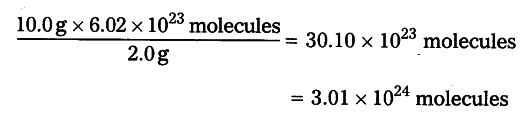# Calculate the volume, mass and number of molecules of hydrogen

Calculate the volume, mass and number of molecules of hydrogen liberated when 230 g of sodium reacts with excess of water at STP. (atomic masses of Na = 23U, O = 16 U and H = 1U)

As per the balanced equation
46 g of of Na gives 2 g of hydrogen.
230 g of Na gives------ ? g of hydrogen.
230g x 2g / 46 g = 10g of Hydrogen.
1 gram molar mass of any gas at STP i.e., standard temperature 273 K and standard pressure 1 bar, occupies 22.4 litres known as gram molar volume.
2.0 g of hydrogen occupies 22.4 litres at STP.
10.0 g of hydrogen occupies------ ? litres at STP.
10.0 g x 22.4 litres / 2.0 g = 112 litres
2 g of hydrogen i.e., 1 mole of H2 contains 6.02 x {{10}^{23}} (H molecules 10 g of hydrogen contain ?